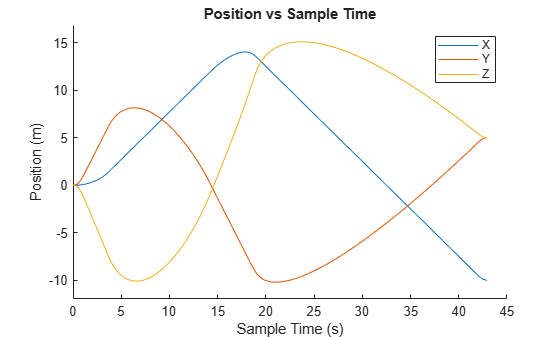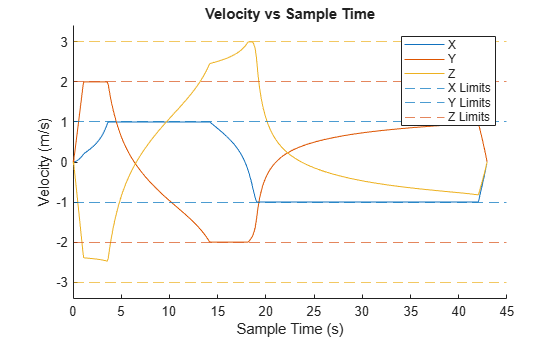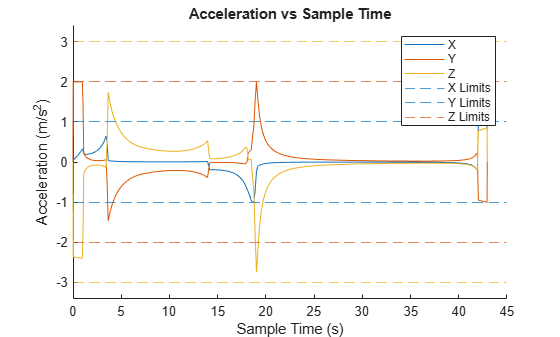# contopptraj

Generate trajectory subject to kinematic constraints

Since R2022b

## Syntax

``[q,qd,qdd,t] = contopptraj(waypoints,vellim,accellim)``
``[___] = contopptraj(polypath,vellim,accellim)``
``[___] = contopptraj(___,NumSamples=N)``
``[___,solninfo] = contopptraj(___)``

## Description

example

````[q,qd,qdd,t] = contopptraj(waypoints,vellim,accellim)` generates a trajectory by fitting a path to a set of waypoints `waypoints`. The function returns a time-optimal trajectory along the path for position `q`, velocity `qd`, and acceleration `qdd` at sample times `t`, while constrained by the velocity `vellim` and acceleration limits `accellim`. This function requires Optimization Toolbox™.```
````[___] = contopptraj(polypath,vellim,accellim)` generates a trajectory along the specified polynomial path `polypath` using all output arguments from the previous syntax.```
````[___] = contopptraj(___,NumSamples=N)` specifies the number of samples to use when generating the trajectory, in addition to any combination of arguments from previous syntaxes.```
````[___,solninfo] = contopptraj(___)` outputs solution information `solninfo` with diagnostic information associated with the output trajectory, in addition to any combination of arguments from previous syntaxes.```

## Examples

collapse all

Create waypoints, velocity limits, and acceleration limits.

```waypoints = [0 5 10 -10; 0 8 -10 5; 0 -10 15 5]; velLimits = [-1 1; -2 2; -3 3]; accelLimits = [-1 1; -2 2; -3 3];```

Generate the position, velocity, acceleration, and time vector of the trajectory with 200 samples.

`[q,qd,qdd,t] = contopptraj(waypoints,velLimits,accelLimits,NumSamples=200);`

Plot the positions, velocities, and accelerations against the sample times `t`.

```figure title("Position vs Sample Time") ylim("padded") hold on q = real(q); qd = real(qd); qdd = real(qdd); t = real(t); plot(t,q(1,:)) % X Position plot(t,q(2,:)) % Y Position plot(t,q(3,:)) % Z Position xlabel("Sample Time (s)") ylabel("Position (m)") legend(["X","Y","Z"]) hold off``````helperPlotConstrainedTrajectory(qd,t,velLimits,"Velocity") ylabel("Velocity (m/s)")``````helperPlotConstrainedTrajectory(qdd,t,accelLimits,"Acceleration") ylabel("Acceleration (m/s^2)")```## Input Arguments

collapse all

Trajectory waypoints for path fitting, specified as an m-by-n matrix. n is the number of elements in the state space, and p is the number of distinct waypoints.

Example: `[1 1 2 3; 2 5 4 5; 5 7 6 8]` is a set of four waypoints with three state space elements each.

Minimum and maximum velocity limits of the trajectory, specified as an n-by-2 matrix, in units per second. n is the number of elements in the state space, and each row is of the form [minimumLimit maximumLimit], specifying the minimum and maximum velocity for each state space element. To generate a trajectory without velocity limits, specify this argument as an empty array.

Example: `[-1 1; -2 5; -5 8]` is a set of velocity limits for three state space elements.

Minimum and maximum acceleration limits of the trajectory, specified as an n-by-2 matrix, in units per seconds squared. n is the number of elements in the state space, and each row is of the form [minimumLimit maximumLimit], specifying the minimum and maximum velocity for each state space element. To generate a trajectory without acceleration limits, specify this argument as an empty array.

Example: `[-1 1; -2 5; -5 8]` is a set of acceleration limits for three state space elements.

Piecewise polynomial path for trajectory generation, specified as a structure such as the output returned from the `mkpp` or `spline` functions. For more information on the fields of this structure, see the `pp` argument of the `mkpp` the function.

Data Types: `struct`

Number of samples for trajectory generation, specified as a positive integer.

Example: `NumSamples=200`

## Output Arguments

collapse all

Trajectory positions, returned as a n-by-m matrix. n is the number of elements in the state space, and m is the number of samples in the trajectory.

Trajectory velocities, returned as a n-by-m matrix. n is the number of elements in the state space, and m is the number of samples in the trajectory.

Trajectory accelerations, returned as a n-by-m matrix. n is the number of elements in the state space, and m is the number of samples in the trajectory.

Sample times, returned as a m-element vector, in seconds. m is the number of samples in the trajectory.

Solution information, specified as a structure containing these fields:

• `ExitFlag` — Number indicating whether `contopptraj` could find a solution. A value of `1` indicates the function successfully found a solution, and a value of `0` indicates that the function was not able to find a feasible solution.

 Pham, Hung, and Quang-Cuong Pham. “A New Approach to Time-Optimal Path Parameterization Based on Reachability Analysis.” IEEE Transactions on Robotics, 34, no. 3 (June 2018): 645–59. https://doi.org/10.1109/TRO.2018.2819195.